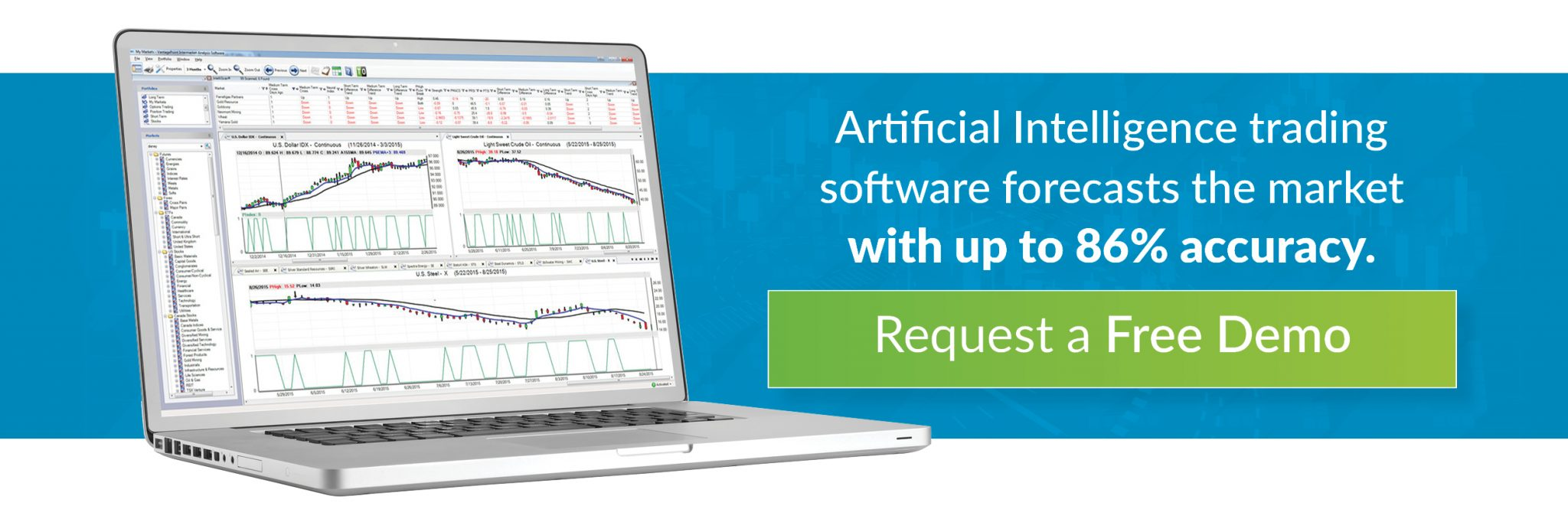Newbie traders and even seasoned investors with a fundamental bias often become overwhelmed by the technicals. But before you develop “analysis paralysis” it’s important to remember that most technical indicators are neither too complex nor too sophisticated for the average retail trader to use.  Watch this short video for an overview of the indicators available in VantagePoint.

### Market Analysis Tools – What is an Indicator?

Let’s begin by clarifying what an indicator is and is not. It is a calculation plotted on or below price action on a chart. This provides an alternate view for interpreting market moves, a view that might not be as obvious when looking at price movement alone. Indicators are used in two main ways: to confirm price movement and the quality of chart patterns, and to form buy and sell signals.  An indicator does not involve random estimations or judgments.

### What is a Moving Average – Chart Analysis Basics

Moving averages (MAs) are one of the most popular and widely used technical indicators. Moving averages smooth the price data to form a trend following indicator. They do not predict price direction, but rather define the current direction with a lag. Moving averages lag because they are based on past prices. Despite this lag, moving averages help smooth price action and filter out the noise. They also form the building blocks for many other technical indicators and overlays, such as Bollinger Bands and the MACD.

The two most popular types of moving averages are the Simple Moving Average (SMA) and the Exponential Moving Average (EMA). These moving averages can be used to identify the direction of the trend or define potential support and resistance levels.

### Simple Moving Averages

An SMA is figured using the closing prices for a specified period, such as 6 days.  If prices are closing lower, the SMA points down. If prices are closing higher, the SMA points up. Plotting this average on a chart could make it much easier to identify the market direction and spot trends, compared to a chart that just shows the open, high, low and close of the price chart’s time frame.

You don’t need complex math to compute an SMA. Actually, they are very simple to figure. Let’s say one is trying to calculate the 6-day moving average of closing prices. Add up the last 6 days and divide the total by 6 to determine the moving average.

The average “moves” because every day the oldest day is dropped off as the current day’s information is added.While the math is seemingly simple, this technical tool is widely available in all standard charting software packages. There will usually be a function to change the parameter of periods, such as a 20-day or 10-hour (depending on chart time frame) for the moving average.

### Limitations of a Simple Moving Average

A criticism of the SMA concept is that each day’s action carries equal weight. In addition, because of the way it is constructed, the SMA trading signals are lagging, not leading, indicators. That means they are only using past data and have no predictive value as to what may happen in the future.
Because of this lagging effect and the desire for traders to have a leading indicator, VantagePoint’s team spent years researching and millions of dollars to create a Predicted Moving Average (PMA).

A 6-day PMA of closing prices takes the past four days of closes, adds two days of predicted data, and then divides that total by six.Six days are still averaged, but day five and day six are predicted. This minimizes, if not totally eliminates, the lag.

### Exponential Moving Averages

Exponential moving averages (EMAs) are similar to SMAs except that more weight is given to the latest data. The weighting applied to the most recent price depends on the number of periods in the moving average. There are three steps to calculating an exponential moving average. First, calculate the simple moving average. An exponential moving average (EMA) has to start somewhere so a simple moving average is used as the previous period’s EMA in the first calculation. Second, calculate the weighting multiplier. Third, calculate the exponential moving average.

The formula below is for a 10-day EMA

SMA: 10 period sum / 10

Multiplier: (2 / (Time periods + 1) ) = (2 / (10 + 1) ) = 0.1818 (18.18%)

EMA: {Close – EMA(previous day)} x multiplier + EMA(previous day).

A 10-period exponential moving average applies an 18.18% weighting to the most recent price. A 10-period EMA can also be called an 18.18% EMA. A 20-period EMA applies a 9.52% weighing to the most recent price (2/(20+1) = .0952). Notice that the weighting for the shorter time period is more than the weighting for the longer time period. In fact, the weighting drops by half every time the moving average period doubles.

Still, even with the weighting of the EMA, it is still a lagging indicator. Only technical tools like VantagePoint Trading Software’s PMA are leading indicators that predict market behavior.

Moving averages can be a useful tool for those looking to identify what type of trend the market is in and when a turning point has occurred.

Again, traders will never catch exact tops or bottoms with moving averages, as they are lagging indicators. However, an indicator like VantagePoint’s Predicted Moving Average can take your technical analysis to a whole different level and literally put you days ahead of other traders.